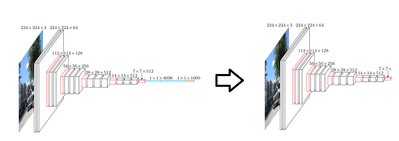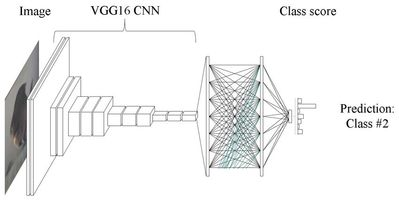# Community Articles

Find and share helpful community-sourced technical articles.

## Deep Learning in Medicine: Classifying Melanoma Part 2: Implementing with TensorFlow and Keras (Steve Kiaie)

Labels (2)

• ### Tensorflow

skiaieCloudera Employee

In part 1 of, we looked at how we could use deep learning to classify Melanoma, using transfer learning with a pre-trained VGG16 convolutional neural network. Here we take a look at how to implement this using TensorFlow and Keras.

First we import the packages we need:

```import numpy as np
from keras.preprocessing.image import ImageDataGenerator
from keras.models import Sequential
from keras.layers import Dropout, Flatten, Dense
from keras import applications```

These packages include, the network layers we will be using, the applications (which includes the pretrained VGG16 model), and some image pre-processing utilities.

Next, we “strip” our model of it’s top layers. This is as simple as one line of code in Keras.

```base_model = applications.VGG16(include_top=False,
weights='imagenet')```Next we take our images of skin, and we transform them using Keras image pre-processing utility:

```datagen = ImageDataGenerator(
rotation_range=40,
width_shift_range=0.2,
height_shift_range=0.2,
rescale=1./255,
shear_range=0.2,
zoom_range=0.2,
horizontal_flip=True,
fill_mode='nearest')```

This will provide us with extra variation in our training image data-set, and will help prevent overfitting in our model.

Next, we feed our processed images through our “stripped” VGG16 network, and generate a feature set.

``` generator = datagen.flow_from_directory(
validation_data_dir,
target_size=(img_width, img_height),
batch_size=batch_size,
class_mode=None,
shuffle=False)

bottleneck_features_validation = model.predict_generator(
generator, nb_validation_samples // batch_size)```

Next we take build a fully connected classifier network.

``` top_model = Sequential()
top_model.compile(optimizer='rmsprop',
loss='binary_crossentropy', metrics=['accuracy'])```

We train this classifier by feeding the feature set generated from our stripped VGG16 network through it. We’ll also save the weights of the trained classifier, once we’ve trained it.

```    top_model.fit(train_data, train_labels,
epochs=epochs,
batch_size=batch_size,
validation_data=(validation_data, validation_labels))
top_model.save_weights(top_model_weights_path)```

Finally, we plug our classifier model back to our stripped VGG16 model, and we re-train the convolutional weights of the VGG16 network.```model = Model(inputs=base_model.input, outputs=top_model(base_model.output))
model.compile(loss='binary_crossentropy',
optimizer=optimizers.SGD(lr=1e-4, momentum=0.9),
metrics=['accuracy'])
model.fit_generator(
train_generator,
steps_per_epoch=nb_train_samples // batch_size,
epochs=epochs,
validation_data=validation_generator,
validation_steps=nb_validation_samples // batch_size,
verbose=2)

```

In this case, our train_generator, and validation_generator’s are created in the same way we created the generator for our classifier.

You can see the full example, and build this for yourself on github, at:

https://github.com/hortonworks-sk/HDP-3.0-classifying-melanoma

1,366 Views
Take a Tour of the Community
Community Browser
Don't have an account?